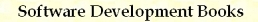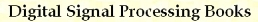Bits and Flags C/C++ Macros
by Stanislav Sýkora, Extra Byte, Via R.Sanzio 22C, Castano Primo, Italy 20022
in Stan's Library, Ed.S.Sykora, Vol.I. First release April 15, 2004.
Permalink via DOI:  10.3247/SL1Soft06.003
 PREVIOUS | NEXT | Code snippets | Software Books and Links Programming Section of Stan' LIBRARY | Stan's HUB
 This document is a part of a collection of macros. For introductory remarks, see the first part (General Purpose Macros). For a review of macro-syntax rules and related tips and tricks, see Writing Macros. For the typedefs DWORD, WORD, BYTE and CHAR, click here.
 CATEGORY MACRO BITS MANAGEMENT mBits, mBitsExcept, mTestBits, mTestBitsExcept mBitsOn, mBitsOnExcept, mBitsOff, mBitsOffExcept mBitsOnOff, mBitsOnOffExcept, mBitsToggle, mBitsToggleExcept FlagArrays mFlagArraySize, mFlagArrayReset mTestFAF, mSetFAF, mResetFAF, mToggleFAF
 Bits Management Code: #define mBits(x,m) ((x)&(m)) #define mBitsExcept(x,m,be) (((x)&(m))& ~(be)) #define mTestBits(x,m,b) (mBits(x,m)==(b))) #define mTestBitsExcept(x,m,b,be) (mBitsExcept(x,m,be)==(b)) #define mBitsOn(lvx,by) ((lvx)|=(by)) #define mBitsOnExcept(lvx,by,be) ((lvx)|=(by)&~(be)) #define mBitsOff(lvx,bn) ((lvx)&= ~(bn)) #define mBitsOffExcept(lvx,bn,be) ((lvx)&= ~((bn)&~(be))) #define mBitsToggle(lvx,b) ((lvx)^= (b)) #define mBitsToggleExcept(lvx,b,be) ((lvx)^= ((b)&~(be))) #define mBitsOnOff(lvx,by,bn) {mBitsOff(lvx,bn);mBitsOn(lvx,by);} #define mBitsOnOffExcept(lvx,by,bn,be) {mBitsOffExcept(lvx,bn,be);mBitsOnExcept(lvx,by,be);} Bits management macros are typically used with bit flagwords in which every bit stands for a true/false flag. On 32-bit platforms, the most efficient usage of these macros consists in reserving a DWORD variable for up to 32 different flags (even when the number of flags to manage is as small as 2, using a whole DWORD still leads to the best performance). Arguments: x is the main operand value. lvx is the main operand left-value; the macros which contain lvx assign a new value to it and also return the new value. m is a bit mask, b any combination of bit flags, by (yes/on bits), bn (not/off bits), be (except bits) may contain any bit pattern All the arguments shauld be of an integer type. In any case, they must be of a type which admits the bitwise operations & (AND), | (OR), ^ (XOR) and ~ (Complement). On 32-bit platforms and using standard integer types, the macros shall be always executed in 32-bit registers and return 32-bit values (DWORD or LONG). When the original width of any of such arguments is smaller than 32 bits, it is automatically cast to 32 bits. This might create problems since such casting fills the extra upper bits with 0's in the case of unsigned integers (or signed positive ones) and with 1's in the case of negative signed integers. In order to avoid such ambiguity, it is best to cast all signed integer arguments whose width is less than 32 bits to a DWORD [for example, mBits(dwArg,(DWORD)signedshortArg)]. mBits(x,m) extracts from x the bits specified by the bit mask m. Use it to tests whether any of the mask bits is set. mBitsExcept(x,m,be) extracts from x the bits specified by the bit mask m, except those which are set in be. mTestBits(x,m,b) extracts from x the bits specified by the bit mask m and compares the result with the bit pattern b. This is equivalent to the expression (mBits(x,m)==(b)). Use this macro to test whether the masked bits coincide with a particular bit pattern. Special case: The call mTestBits(x,m,m) returns true only if all the bits set in m are also set in x. mTestBitsExcept(x,m,b,be) is equivalent to the expression (mBitsExcept(x,m,be)==(b)). Use it to test whether the masked bits of x, except those which are set in be, coincide with a particular bit pattern. mBitsOn(lvx,by) sets to 1 those bits of lvx which are set in b, leaving unchanged all others. Use it to simultaneously set to 1 several flags in a bit-coded flagword. mBitsOnExcept(lvx,by,be) sets to 1 those bits of lvx which are set in b but not in be, leaving unchanged all others. mBitsOff(lvx,bn) sets to 0 those bits of lvx which are set in b, leaving unchanged all others. Use it to simultaneously clear (reset to 0) several flags in a bit-coded flagword. mBitsOffExcept(lvx,bn,be) sets to 0 those bits of lvx which are set in b but not in be, leaving unchanged all others. mBitsToggle(lvx,b) toggles those bits of lvx which are set to 1 in b, leaving unchanged all others (the toggle operation is the same as XOR with 1). Use this macro to simultaneously toggle several flags in a bit-coded flagword. mBitsToggleExcept(lvx,b,be) toggles those bits of lvx which are set in b but not in be, leaving unchanged all others. mBitsOnOff(lvx,by,bn) is equivalent to executing mBitsOff(lvx,bn) and then mBitsOn(lvx,by). mBitsOnOffExcept(x,b,be) is equivalent to executing mBitsOffExcept(x,bn,be) and then mBitsOnExcept(x,by,be).
 FlagArrays Management Code: #define mFlagArraySize(nflags) (((nflags)+31)>>5) #define mFlagArrayReset(lpFA,nflags,n) {for (n=0;n>5] & (1<<((n)&0x1f))) #define mSetFAF(lpFA,n) ((lpFA)[(n)>>5] |= (1<<((n)&0x1f))) #define mResetFAF(lpFA,n) ((lpFA)[(n)>>5] &= ~(1<<((n)&0x1f))) #define mToggleFAF(lpFA,n) ((lpFA)[(n)>>5] ^= (1<<((n)&0x1f))) When you need to manipulate a large number of flags (say 1000), pack them into an array of DWORDs, each containing up to 32 flags (one per bit). The following macros simplify operations on individual flags in such a FlagArray. Arguments: nflags (DWORD) is the total number of flags to handle lpFA (DWORD*) points to a FlagArray n (DWORD) specifies the n-th flag (bit) of a FlagArray mFlagArraySize(nflags) returns the number of DWORDs required to contain the specified number of flags. For examples of use, see the next macro. mFlagArrayReset(lpFA,nflags,n) clears all flags in the FlagArray. In this case, n is just an auxiliary integer variable and the macro does not return any significant value. Examples of use: (a) Dynamic allocation: DWORD n; DWORD* lpFA = new DWORD[mFlagArraySize(nflags)]; mFlagArrayReset(lpFA,nflags,n)]; ...; // do anything here, using all the other ...; // macros to manipulate individual flags delete [] lpFA; // Do not forget to disallocate   (b) Static allocation: DWORD n; DWORD lpFA[mFlagArraySize(100000)]; mFlagArrayReset(lpFA,nflags,n)]; ...; // do anything here, using all the other ...; // macros to manipulate individual flags mFlagArrayReset(lpFA,nflags,n) clears all flags in the FlagArray. In this case, n is just an auxiliary integer variable and the macro does not return any significant value. Examples of use: (a) Dynamic allocation: DWORD n; DWORD* lpFA = new DWORD[mFlagArraySize(nflags)]; mFlagArrayReset(lpFA,nflags,n)]; ...; // do anything here, using all the other ...; // macros to manipulate individual flags delete [] lpFA; // Do not forget to disallocate   (b) Static allocation: DWORD n; DWORD lpFA[mFlagArraySize(100000)]; mFlagArrayReset(lpFA,nflags,n)]; ...; // do anything here, using all the other ...; // macros to manipulate individual flags mTestFAF(lpFA,n) tests the n-th flag of the FlagArray and return 1 when set or 0 when not set. mSetFAF(lpFA,n) sets the n-th flag of the FlagArray to 1. mResetFAF(lpFA,n) resets the n-th flag of the FlagArray to 0. mToggleFAF(lpFA,n) toggles the n-th flag of the FlagArray.TOP | PREVIOUS | NEXT | Code snippets | Software Books and Links Programming Section of Stan' LIBRARY | Stan's HUB
 Copyright ©2004,2006 Stanislav Sýkora    DOI: 10.3247/SL1Soft06.003 Designed by Stan Sýkora